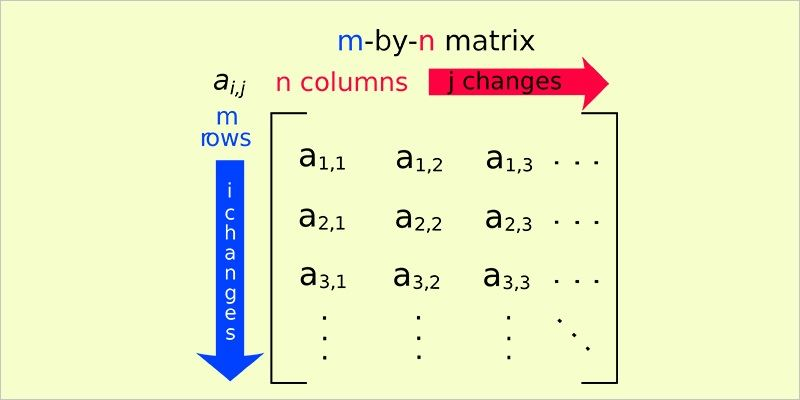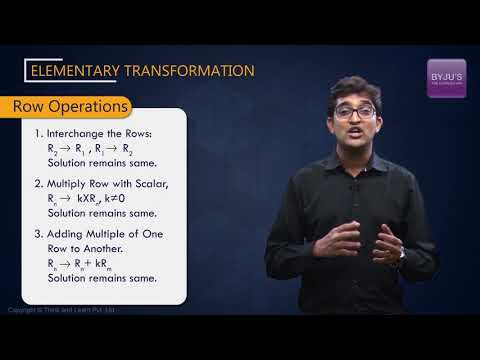# Elementary Transformation Of Matrices

We have already seen that two matrices are equal when they are of the same order and their corresponding elements are equal. That is, if P = [pij]m×n  and Q = [qij]r×s are two matrices such that P = Q, then:

• m = r and n = s i.e. the orders of the two matrices must be the same
• For every value of i and j, pij = qij.

But there is another way to compare matrices. In this case, the matrices are equivalent to each other. For a matrix, P = [pij]m×n to be equivalent to a matrix Q = [qij]r×s, i.e. P ~ Q , the following two conditions must be satisfied:

• m = r and n = s; again, the orders of the two matrices must be the same
• P should get transformed to Q using the elementary transformation and vice-versa.

Elementary transformation of matrices is very important. It is used to find equivalent matrices and also to find the inverse of a matrix. Elementary transformation is playing with the rows and columns of a matrix. Let us learn how to perform the transformation on matrices.## Elementary Row Transformation

As the name suggests, only the rows of the matrices are transformed and NO changes are made in the columns. These row operations are executed according to a certain set of rules which make sure that the transformed matrix is equivalent to the original matrix. These rules are:

Rule 1: Any two rows are interchangeable. The interchange of ith and jth rows is represented as:

Ri ↔ Rj

For example: Let

$$\begin{array}{l}A = \begin{bmatrix}1 & 23\cr-9 & 5\cr-13 & 7\end{bmatrix}\end{array}$$

Applying R1 ↔ R3, we get;

$$\begin{array}{l}B =\begin{bmatrix}-13 & 7\cr-9 & 5\cr1 & 23\end{bmatrix}\end{array}$$

Here, A ~ B.

Rule 2: All the elements of any row can be multiplied by any non-zero number. The multiplication of ith row to a non-zero number k, is represented as:

Ri → kRi

For example: Let,

$$\begin{array}{l}A = \begin{bmatrix}1 & 6 & 8\cr3 & 5 & 21\cr2 & 1 & 9\end{bmatrix}\end{array}$$

Applying R2 2R2, we get;

$$\begin{array}{l}B = \begin{bmatrix}1 & 6 & 8\cr6 & 10 & 42\cr2 & 1 & 9\end{bmatrix}\end{array}$$

Again, A ~ B.

Rule 3: All the elements of a row can be added to corresponding elements of another row multiplied by any non-zero constant. If the elements of ith row are being added to the elements of  jth row (multiplied by a non-zero number k), then it is represented as:

Ri → Ri + k Rj

An important thing to note here is that the change will only reflect in the elements of ith row. The elements of jth row will remain same.

For example: Let,

$$\begin{array}{l}A = \begin{bmatrix} 4&10 &64 \\ 1& -13 & 9 \\2 & 5 & 32 \\\end{bmatrix}\end{array}$$

Applying R1 R1 + (-2)R3 , we get,

$$\begin{array}{l}\begin{bmatrix}0 & 0 & 0 \\1 & -13 & 9 \\2 & 5 & 32 \\\end{bmatrix}B =\end{array}$$

Here,  A ~ B

It’s easy to figure out here that if k = 1, it just reduces to adding the elements of ith row to corresponding elements of jth row. Also, if k = -1, it means subtracting the elements of jth row from the corresponding elements of the ithrow.

For example: Let

$$\begin{array}{l}P = \begin{bmatrix}1 & 5 & 4 \\5 & 2 & 5 \\4 & 5 & 3 \\\end{bmatrix}\end{array}$$

Applying R2 R2 + R3, we get,

$$\begin{array}{l}Q = \begin{bmatrix}1 & 5 & 4 \\9 & 7 & 8 \\4 & 5 & 3 \\\end{bmatrix}\end{array}$$

P ~ Q

Those were the three elementary row transformations/operations. The elementary column operations are exactly the same operations done on the columns.

## Elementary Column Operation

Those three operations for rows, if applied to columns in the same way, we get elementary column operation. As we have already discussed row transformation in detail, we will briefly discuss column transformation. The rules for elementary column transformation are:

Rule 1: Any two columns are interchangeable i.e., Ci Cj.

For example: Let,

$$\begin{array}{l}A =\begin{bmatrix}1 & 23\cr-9 & 5 \cr-13 & 7\end{bmatrix}\end{array}$$

Applying C1 ↔ C2, we get;

$$\begin{array}{l}B =\begin{bmatrix}23 & 1\cr5 & -9\cr7 & -13\end{bmatrix}\end{array}$$

Here,  A ~ B.

Rule 2: All the elements of any column can be multiplied by any non-zero number i.e., Ci ↔ k Ci.

For example: Let,

$$\begin{array}{l}A =\begin{bmatrix}1 & 6 & 8\cr3 & 5 & 21\cr2 & 1 & 9\end{bmatrix}\end{array}$$
.

Applying C2 3 C2, we get,

$$\begin{array}{l}B =\begin{bmatrix}1 & 18 & 8 \cr3 & 15 & 21\cr2 & 3 & 9\end{bmatrix}\end{array}$$

Again,  A ~ B

Rule 3: All the elements of a column can be added to corresponding elements of another column multiplied by any non-zero constant i.e., Ci → k Cj.

For example: Let,

$$\begin{array}{l}A = \begin{bmatrix}4 & 10 & 1\cr1 & -13 & 1\cr2 & 5 & 2\end{bmatrix}\end{array}$$

Applying C1 C1 + 3C3, we get,

$$\begin{array}{l}B= \begin{bmatrix}7 & 10 & 1\cr4 & -13 & 1\cr8 & 5 & 2\end{bmatrix}\end{array}$$

Here,  A ~ B .

Those were the elementary transformation techniques for matrices. As mentioned before, these are very important and are used to find the inverse of a matrix. To know how to find the inverse of a matrix, visit www.byjus.com and learn the concepts, explained in a very easy way.## Frequently Asked Questions – FAQs on Elementary Transformation of Matrices

Q1

### What is Elementary Transformation of Matrices?

Elementary Transformation of Matrices means playing with the rows and columns of a matrix. Or operations are done on the rows and columns of matrices to change their shape so that the computations become easier.

Example:- Row → R and Column → C

If A = [1 2]

Now perform, C1 → C2 ↔ C1
So, A = [2 1]

Q2

### In the operation of a row, the entire row in a matrix is swapped with another row?

Yes, in the operation of a row, the entire row in a matrix is swapped with another row.
Q3

### Can we multiplied row or column with the same non zero number?

Yes, the entire row or column is multiplied with the same non zero number.
Q4

### Can we use column operations in elementary transformation?

Elementary transformation of matrices is very important. It is used to find equivalent matrices and also to find the inverse of a matrix.
So, yes we can use column operations in elementary transformation.